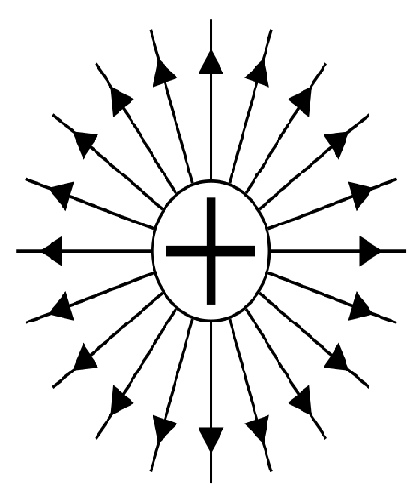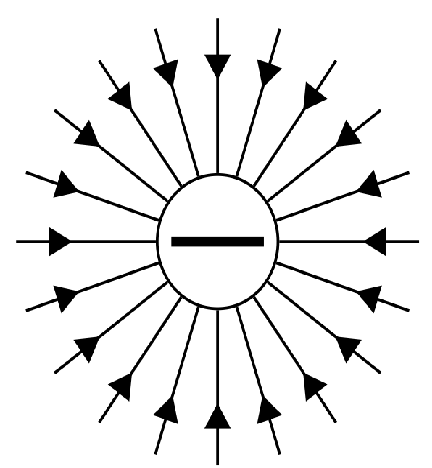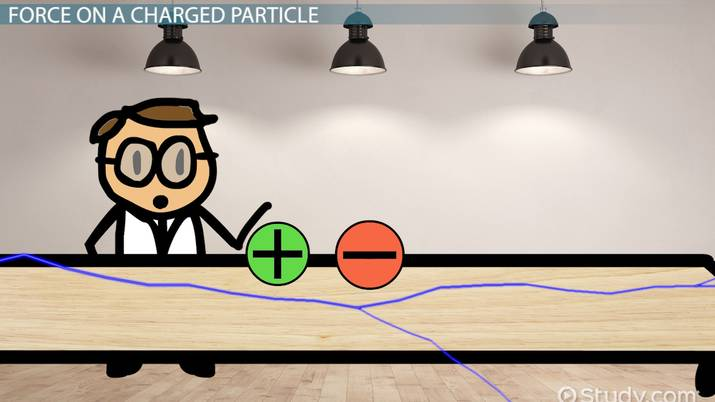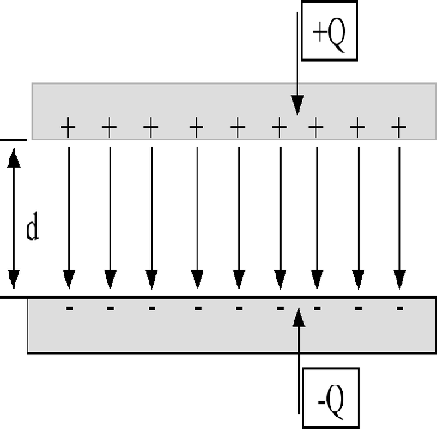# Electric Field Between Two Plates: Formula & Calculations

Rashid Nuzha, Betsy Chesnutt, Elaine Chan
• Author
Rashid Nuzha

Rashid has earned a Postgraduate Certificate in Professional Studies in Education (PGCPSE) in 2021 from The Open University-United Kingdom. He obtained ITTT 120-hour TEFL Certificate in 2019 and has held a BSc in Physics and Mathematics since 2005. Also, Rashid has 7-years of experience working in an international school as a teacher and administrator.

• Instructor
Betsy Chesnutt

Betsy has a Ph.D. in biomedical engineering from the University of Memphis, M.S. from the University of Virginia, and B.S. from Mississippi State University. She has over 10 years of experience developing STEM curriculum and teaching physics, engineering, and biology.

• Expert Contributor
Elaine Chan

Dr. Chan has taught computer and college level physics, chemistry, and math for over eight years. Dr. Chan has a Ph.D. in Chemistry from U. C. Berkeley, an M.S. Physics plus 19 graduate Applied Math credits from UW, and an A.B. with honors from U.C .Berkeley in Physics.

Learn about the electric field between parallel plates, and its direction. Use the formula for the electric field between two plates to calculate its magnitude. Updated: 12/20/2021

Show

## Electric Field of a Plate

A charged particle carries either negative or positive charges. A negatively charged particle carries more electrons (negative charges) than protons (positive charges) since electrons are always negatively charged. So, for charged particles to be positive, fewer electrons should exist compared to the number of protons. Charged particles placed on a plate create a positively or negatively charged plate, and a charged plate creates an electric field around it. An electric field is an area or space around charged particles or objects where the influences of an electric force on other charged particles or objects are visible.Carl Gauss, a famous physicist, studied the electric field flux through closed surfaces. Gauss investigated charged particles by considering them spheres in space. In general terms, Gauss's law states that the electric field flux through a closed surface is the product of the surface's area by the electric field vector standing perpendicular to the surface's area. Gauss's law depends on the surface's shape. For geometrically defined shapes, calculating the area becomes an easy task. In the case of a sphere or rectangular plate, Gauss's law equation can be given as the surface's charge density divided by the electric permittivity of the medium.An error occurred trying to load this video.

Try refreshing the page, or contact customer support.

Coming up next: Electric Potential Energy: Definition & Formula

### You're on a roll. Keep up the good work!

Replay
Your next lesson will play in 10 seconds
• 0:04 Force on a Charged Particle
• 1:04 Magnitude & Direction of Field
• 2:48 Motion of a Charged Particle
• 4:34 Lesson Summary
Save Save

Want to watch this again later?

Timeline
Autoplay
Autoplay
Speed Speed

## How to Create an Electric Field between the two Parallel Plates?

Place two conducting plates A and B parallel to each other while leaving a small space between them filled with an electrical insulator such as air. Connect the two parallel plates to an electric power source ( a battery, for example). Plate A, which is connected to the positive pole of the power source, will be positively charged with a uniform charge density +Q. In contrast, plate B, which is connected to the negative pole of the power source, will be negatively charged with a consistent charge density -Q. The two charged parallel plates would carry their total charges because an electric insulator separates them.

The two parallel charged plates are kept apart and create a uniform electric field in the space between them. The electric field lines of two parallel plates can be represented by straight lines perpendicular to both plates' surfaces while carrying arrows that point from +Q plate A toward -Q plate B.On the one hand, the electric field between two parallel plates depends on the charge's density and the medium permittivity according to Gauss's law. On the other hand, according to Coulomb's law, the same electric field between two parallel conducting plates depends on the electric potential or voltage of the two plates and the distance between the two plates.

So, the electric field {eq}E=\frac{V}{d} {/eq} where d is the distance between the two charged plates. It is clear that the electric field of two parallel and oppositely charged plates is inversely proportional to the distance, so when the two plates are brought closer, therefore, the distance (d) between the plates decreases, which results in the electric field increasing. The uniformly and oppositely charged two parallel plates store electric potential energy due to the difference in the number of charges carried on both conducting plates.

In two parallel but like-charged plates, the electric field in the space between plates is equal to zero. In this case of both positively or negatively charged and parallel plates, the charges repel each other, creating two oppositely directed electric fields in the space in-between the two plates. Therefore, the two like-charged plates defy the purpose of using the system of two charged parallel plates as electric potential energy storage.

### Electric Field Between Two Plates: Formula for Magnitude

Assuming that two parallel conducting plates carry opposite and uniform charge density, the formula can calculate the electric field between the two plates: {eq}E=\frac{V}{d} {/eq}, where

V is the electric potential or voltage measured by volts (V)

d is the distance between the two plates measured by meters (m)

E is the electric field measured by N/m

#### Example 1

Calculate the electric field between two oppositely charged plates with an electric potential of 1.5 volts and a distance of 1.0 cm. If the two plates are moved to 2.0 cm, what happens to the magnitude of the electric field of the two parallel plates?

Solution:

Remember to always convert all numbers to SI units.

d=1 cm={eq}1.0*10^{-2} m {/eq} m

E=V/d

{eq}E=\frac{1.5}{1.0*10^{-2}} {/eq}

E={eq}1.5*10^{2} m {/eq} N/m (two significant figures)

For this example, imagine a battery of 1.5 V is connected to two parallel plates that are kept apart at a small distance of 1 cm; the electric field is 150 N/m, a large magnitude of an electric field because of the small distance of 1 cm.

When d= 2.0 cm= {eq}2.0*10^{-2} m {/eq} then

{eq}E=\frac{1.5}{2.0*10^{-2}} {/eq}

{eq}E=7.5*10^{1} {/eq} N/m (two significant figures)

To unlock this lesson you must be a Study.com Member.

• Activities
• FAQs

## Electric Fields from 3 Parallel Plates

This problem has been given to help you understand superposition of electric fields.

The electric field from a thin conducting large plate is Ei = qi / (2Ae_0) in direction outward, from each side of the plate. We assume positive charge in the formulas. For negative charge the direction is inward, toward the plate.

• qi is the charge in coulombs.
• A is area in meters squared.
• e_0 is a constant equal to 8.85 × 10^-12 C^2/Nm^2.

All plates have the same area and are large enough so that edge effects are negligible. Consider the first plate with charge q1 lying between regions A and B. The electric field E1 in region A is to the left and to the right in region B and all other regions further right.

Add a plate with charge q2 on the right, in the region between B and C. E2 is pointing to the left in regions A and B and to the right in region C and all points to the right of region C.

Add a third plate of charge q3 in the region between C and D. E3 is pointing to the right in region D and to the left in regions A, B, and C. To visualize and summarize:

• A | B | C | D

We use superposition to add up the electric fields in the four regions.

• In region A the electric field is to the left and equals (q1 + q2 + q3) / (2Ae_0).
• In region B the electric field is to the right (q1 - q2 - q3) / (2Ae_0).
• In region C the electric field is to the right (q1 + q2 - q3) / (2Ae_0).
• In region D, the electric field is to the right (q1 + q2 + q3) / (2Ae_0).

Let's try to calculate with:

• q1 / A = -0.5 × 10^-6 C/m^2
• q2 / A = 0.1 × 10^-6 C/m^2
• q3 / A = -0.35 × 10^-6 C/m^2

What is the electric field in regions B and C?

• In region B the electric field is -1.4 × 10^4 N/C to the right.
• In region C the electric field is -2.8 × 10^3 N/C to the right.

#### What is the electric field between plates?

The electric field between plates is the area or space where the plates' charges influences can be seen. For example, if a charged particle is placed near any charged plate, the plate exerts an electric force to attract or repel the charged particle.

#### Is the electric field between two plates uniform?

If the two parallel plates are oppositely and uniformly charged, then each plate carries an equal charge density allowing the electric field between the two plates to be uniform.

An electric field between two plates needs to be uniform. Therefore, charges must be equally distributed on the two plates.

#### How to find the electric field between two plates?

The electric field between two oppositely charged plates can be calculated: E=V/d.

Divide the voltage or potential difference between the two plates by the distance between the plates. The SI units are V in volts(V), d in meters (m), and E in V/m.

### Register to view this lesson

Are you a student or a teacher?